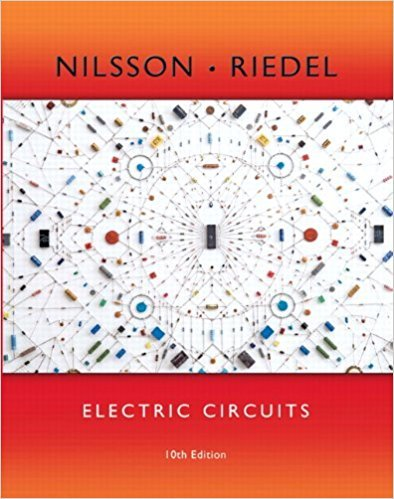×
×

# Solved: Find the average power dissipated in the resistor in the circuit seen in FigISBN: 9780133760033 390

## Solution for problem 10.6 Chapter 10

Electric Circuits | 10th Edition

• Textbook Solutions
• 2901 Step-by-step solutions solved by professors and subject experts
• Get 24/7 help from StudySoup virtual teaching assistantsElectric Circuits | 10th Edition

4 5 1 361 Reviews
18
1
Problem 10.6

Find the average power dissipated in the resistor in the circuit seen in Fig. P10.6 if

Step-by-Step Solution:
Step 1 of 3

l,ACrt (x()= =7f Ynr+ - r- tP) E : Y, + -.: l',Oz pt)T .;h J\. VorCu O ig.^) +N .-\z =- t 14'l, ,ltr 41 /...

Step 2 of 3

Step 3 of 3

##### ISBN: 9780133760033

Since the solution to 10.6 from 10 chapter was answered, more than 218 students have viewed the full step-by-step answer. This textbook survival guide was created for the textbook: Electric Circuits, edition: 10. This full solution covers the following key subjects: . This expansive textbook survival guide covers 107 chapters, and 1357 solutions. The answer to “Find the average power dissipated in the resistor in the circuit seen in Fig. P10.6 if” is broken down into a number of easy to follow steps, and 16 words. The full step-by-step solution to problem: 10.6 from chapter: 10 was answered by , our top Engineering and Tech solution expert on 03/13/18, 07:48PM. Electric Circuits was written by and is associated to the ISBN: 9780133760033.

Unlock Textbook Solution